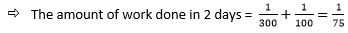# Explanation

Method 1

Let the total work to build fifth floor is 1 unit.

• The efficiency of worker of Team D = 1/100.

The efficiency of worker of Team C = 1/3 * Efficiency of worker of Team D.

• The efficiency of worker of Team C = 1/300

As each worker works alternatively:Thus it will take 75 number of alternate working days of both Team C and Team D to build 5th Floor.

Hence, the total number of days taken to build the 5th Floor is 75*2 = 150 days

Method 2

• The efficiency of worker of Team D = 1/100.

The efficiency of worker of Team C = 1/3 * Efficiency of worker of Team D.

The efficiency of worker of Team C = 1/300

Let the total work required is 300 units [here we have considered LCM of time taken by both the workers i.e. 100 & 300].

Work done by worker of Team C per day = 300/300 = 1 unit.

Work done by worker of Team D per day = 300/100 = 3 units.

AS per the question they are working alternatively.

Work done in 2 days = Work done by worker of Team C + Work done by Team D = 1 unit + 3 units = 4 units.

Total time taken = 300/4 = 75 alternate working days for both the worker = 75*2 = 150 days.